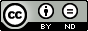### Aboodh Homotopy Perturbation Method of solving Burgers Equation.

Oludapo Omotola Olubanwo, Olutunde Samuel Odetunde, Adetoro Temitope Talabi

#### Abstract

In this paper, we present a reliable combination of Aboodh Transform and Homotopy perturbation method to determine the exact solution of one dimensional Burgers equation which is a nonlinear partial differential equation. Some cases of one dimensional nonlinear partial differential equations are considered to illustrate the capability and reliability of Aboodh Homotopy perturbation method. We have compared the analytical solution obtained with the available Laplace decomposition method. The solution which is found to be exactly the same. The result revealed that the combination of the Aboodh Transform and homotopy perturbation method is quite capable, practically well appropriate for use in such problems. The method is tested on three examples and results show that the new method is more effective and convenient to use and high accuracy of it is evident.

#### Keywords

Aboodh Transform, Homotopy Perturbation method, Burgers equation, Nonlinear Partial differential equation.

PDF

#### References

Rogers, C. and Shadwich, W. F. Backlund Transformations and Their applications, New York: Academic Press, 1982.

Hirota, R.” Exact solutions of the Korteweg-De vries Equation for Multiple collisions of Solitons”. Physical Review Letters, vol. 27, no. 18, pp. 1192-1194, 1971.

He, J. H. “Variational iteration method for autonomous ordinary differential systems” pp. 115-123, 2000.

He, J. H. “Approximate Analytical solution for seepage flow with fractional derivatives in porous media”, Computer Methods in Applied Mechanics and Engineering, vol.167, no.1-2, pp. 57-68, 1998.

Adomian, G. “A Review of the Decomposition Method in Applied Mathematics”, Journal of Mathematical Analysis and Applications, 135, 501-544, 1988.

Liao, S. J. “The proposed homotopy analysis technique for the solution of nonlinear problems”, PhD Thesis, Shanghai Jiao Tong University, 1992.

Liao, S. J. “An explicit, totally analytic approximate solution”, International Journal of Non-Linear Mechanics, vol. 34, pp. 759-778, 1999.

Liao, S. J. “On the homotopy analysis method for nonlinear problems”, Journal of Applied Mathematics and Computation, vol. 147, no. 2, pp. 499-513, 2004.

Liao, S. J. “Series solutions of unsteady boundary-layer ﬂows over a stretching ﬂat plate”, Studies in Applied Mathematics, vol. 117, no. 3, pp.239-263, 2006.

He, J. H. “The homotopy perturbation method for nonlinear oscillators with discontinuities” Journal of Applied Mathematics and computation, vol.151, no. 1, pp. 287-292, 2004.

He, J. H. “Application of homotopy perturbation method”, Journal of Chaos, Solitons and Fractals, vol. 26, pp. 695-700, 2005.

He, J. H. “Homotopy perturbation method: a new nonlinear analytical technique”, Journal of Applied Mathematics and Computations, pp. 73-79, 2003.

He, J. H. “A coupling method of a homotopy technique and a perturbation technique for non-linear problems”, International Journal of Non-Linear Mechanics, vol. 35, pp. 37-43, 2000.

Aboodh, K. S. “The New Integral Transform Aboodh Transform", Global Journal of Pure and Applied Mathematics ISSN0973-1768, vol. 9, no. 1, pp. 35-43, 2013.

Aboodh, K. S. “Application of New "Aboodh Transform" to Partial Differential Equations”, Global Journal of pure and Applied Mathematics, vol. 10, no. 2, pp. 249-254, 2014.

Ahamad J, Bibi Z, Noor K. “Laplace Decomposition Method using He's Polynomial to Burgers Equation” Journal of Science and Arts, vol. 2, no. 27, pp. 131-138, 2014.

Biazar, J. and Ghazvini, H. “Exact Solutions for nonlinear Burgers equation by Homotopy Perturbation method”, Journal of Numerical Methods for Partial Differential Equations, 2008.

DOI: https://doi.org/10.24203/ajas.v7i2.5794

### Refbacks

• There are currently no refbacks.